# MA2051 - Ordinary Differential EquationsSolutions to Review 1

1.
(a)
Just integrate to obtainand use the initial data to find C = 3.

(b)
Separate and integrate (using partial fractions) to obtain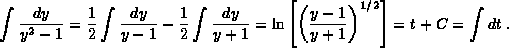Rearrange to findand use the initial data to find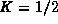. Solve finally for y,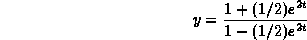and note that this solution is valid only for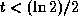if you start at t = 0.

2.
(a)
The balance law behind the equation is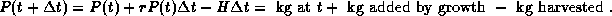Rearrange, divide byand let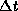go to zero to obtain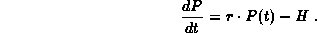The growth rate is equal to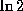divided by the doubling time, so.

(b)
The maximum (constant) harvest rate that does not wipe out the population iswhere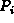is the initial population. You can get this from the solution for the initial value problem or just by setting the righthand side of the differential equation equal to zero. You have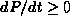, so the population does not decay, if and only if. If, on the other hand,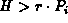, thenfor all time and the yeast population will reach zero. The amount harvested in a day with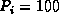kg and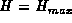is then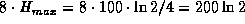, which is approximately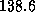kg per day.

(c)
Just let the yeast grow for eight hours and then harvest it all at once. You have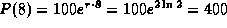kg per day, which is a much larger yield per day than the maximum for constant harvesting.

3.is a homogeneous solution and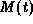is a particular solution so, because the equation is linear, the general solution is. The initial data determine C from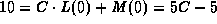, or C= 3.

4.
(a)
The characteristic equation is r + 3 = 0 so a homogeneous solution is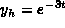.

(b)
Look for a particular solution of the form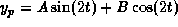. Plug it in to obtain the equations 2A + 3B = 13 and 3A - 2B = 0 for A and B. The solutions are A=2 and B=3.

(c)
Solve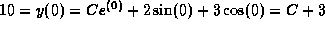and obtain C= 7, hence.

(d)
The exponential term is transient and so the solution looks like the particular solution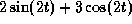for large t.

5.
(a)
Solveto obtain two steady states y=1 and y=3; these are constant solutions for the differential equation.

(b)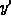is positive and so y is increasing for y<1 and y > 3.is negative and so y is decreasing for 1 < y < 3. The solution starting at 0 increases and approaches y=1. The solution starting at 1 remains y=1. The solution starting at 5 increases and does not approach anything.

(c)
The sketch indicates that y = 1 is stable because trajectories which start nearby converge to y=1. The steady state y=3 is unstable; trajectories starting at any y> 3 increase and do not stay close to 3.

(d)
Use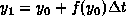with,and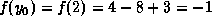. You obtain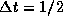(whatever units you want).

These solutions are provided without warranty, implied or otherwise. Use at your own risk.
```

© 1996 by Will Brother.
All rights Reserved. File last modified on March 22, 1996.

```